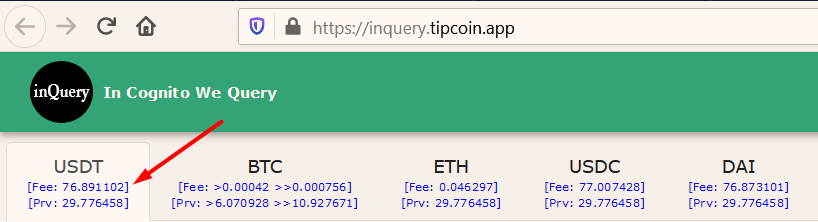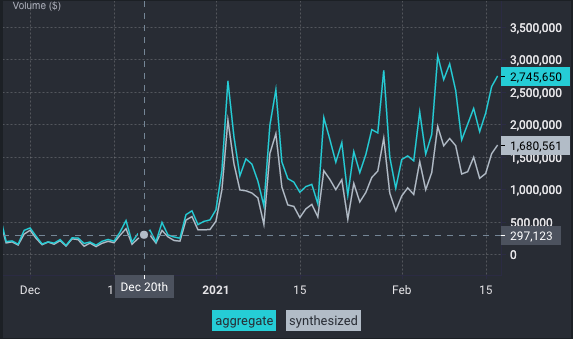# abduraman - InQuery - In Cognito We Query

Hey @abduraman someone from the dev team will answer shortly.

3 Likes

hi @abduraman for current PRV price we calculate direct pool prv/pToken

I write example :
first get pdex state to get pool size via rpc :

``````{
"jsonrpc":"1.0",
"method":"getpdestate",
"params":[{
"BeaconHeight":<current value BeaconHeight>
}],
"id":1
}
``````

example result :

`//Pool Size PRV-pBTC "pdepool-962075-0000000000000000000000000000000000000000000000000000000000000004-b832e5d3b1f01a4f0623f7fe91d6673461e1f5d37d91fe78c5c2e6183ff39696": { "Token1IDStr": "0000000000000000000000000000000000000000000000000000000000000004", "Token1PoolValue": 2794646452014123, "Token2IDStr": "b832e5d3b1f01a4f0623f7fe91d6673461e1f5d37d91fe78c5c2e6183ff39696", "Token2PoolValue": 181299347091 },`

then cal for price pBTC in PRV Price :

``````    var decimal1 int64
var decimal2 int64

decimal1 = 9  // decimal PRV
decimal2 = 9 // decimal pBTC

var Token2PoolValue float64
var Token1PoolValue float64

Token1PoolValue = 2794646452014123 // PRV in pool size
Token2PoolValue = 181299347091  // pBTC in pool size

x2 := math.Ceil((Token2PoolValue *Token1PoolValue) / (Token2PoolValue + math.Pow10(int(decimal2))))
price := (Token1PoolValue - x2) / math.Pow10(int(decimal1))

fmt.Println(price)
``````

https://play.golang.org/p/LMXImgEkRhf

2 Likes

Why do you calculate the price in this way? What is the rationale behind it? @binh

Both incscan and my bots calculate the price just by dividing pool values:

``````price := Token1PoolValue * math.Pow10(-int(decimal1)) / (Token2PoolValue * math.Pow10(-int(decimal2)))
``````

So the results of incscan (any my products) and https://api.incognito.org/ptoken/list are different. To me, the correct one is inscan’s one. However, I want to know whether your code is buggy or has a rationale. Please could you clarify the issue? Or could you point out a link explaining your formula?

Thanks.

2 Likes

hey @abduraman, I just wanted to confirm that the formula @binh mentioned above is correct, so please update yours to that if possible, thanks.

2 Likes

What is the rationale behind it? @duc I cannot update the formula of Network Explorer since I’m not its developerI and @inccry calculate the price with the formula which I give. Could you want to join the discussion @inccry?

2 Likes

ah please have a look at the post as well as Automated Market Maker algorithm to understand the rationale behind it @abduraman

3 Likes

This explains why the deviation between your BTC/PRV index and Network Explorer’s BTC/PRV index is much higher than your ETH/PRV index and Network Explorer’s ETH/PRV index.

Imho @binh’s code has a small bug that does not affect the result. I’ll write it in the end. Let me try to formalize the difference between your calculation and Network Explorer’s calculation.

Let x, y, dx, dy be Token1PoolValue (PRV), Token2PoolValue (BTC), buy amount, and sell amount respectively.

I and Network Explorer calculate the price via `x/y` formula. As per Automated Market Maker algorithm document, you calculate the price via `dx/dy` formula. I confirmed this via the wallet app, too.

However, in your approach, imho there is a problem. For each coin, you assume that the user sells 1 unit of that coin, i.e. 1 BTC, 1 ETH, or 1 USDT etc. Here is the derivation.

``````x * y = (x - dx) * (y + dy)

(x * y) / (y + dy) = x - dx

dx = x  -  (x * y) / (y + dy)

Final formula: dx/dy = ( x  -  (x * y) / (y + dy) ) / dy
``````

Your code replaces `dy` with 1 unit of the corresponding coin in the formula as I wrote. So in fact, the prices in https://api.incognito.org/ptoken/list are the answers to “if the user sold 1 BTC, 1 ETH or 1 USDT, what would be the price?”. I think you will insist on this approach. No problem. I’ll update my code to show the prices compatible with Network Explorer.

Small bug: Since decimal1 and decimal2 have the same value, the results aren’t affected. However, `decimal1` in `price := (Token1PoolValue - x2) / math.Pow10(int(decimal1))` should be `decimal2` too. FYI.

3 Likes

I confirm, I won’t change Network Explorer calculations. It makes no sense on my side to show a price given a fixed bought/sold amount.

Maybe an extra feature for the Network Explorer : tell me how many coins you want to buy/sell and I’ll tell you the amount you’ll receive given the current pool state.

Thanks @abduraman for your great explanation!

4 Likesv1.0.4 releasedUpdate:

• As per the discussion above, the prices become compatible with Network Explorer. So they are much more accurate now.
5 Likes

I saw a post that this project shows unshielding fees without needing to check the wallet. Where is this? I’d find it extremely helpful as I hate the high fees!

Nevermind. I found it. This is very useful!5 Likes

4 Likes

Maybe someone saw that you bought so many (pushing price up) and sold off a few, then you bought PRV back and the price had changed. You can look back at that time and see if that’s the case. Was this the case?

This tool was useful at day 0. Now this is even more helpful! @abduraman and @inccry, does it make sense to cross link between your two products to make it more useful for users to find the other tools? From a user perspective this seems like it would be helpful.

1 Like

Hey @RobynFitzooth,

As for your suggestion, I have a plan to make the main domain (tipcoin.app) a hub for both Tipcoin and Incognito Ecosystem but I need some time.

is that what impermenant loss is? when you are the buy then seller of two immediate transactions and 30% of value poofs into thin are like this?

No.

At a very basic level, impermanent loss is the difference in value between the combined amount of assets someone places into a liquidity pool – at any given time, versus the value of those same assets if just HODLed – at the same time. To be clear, this is a very crude definition that glosses over a lot of detail. However @andrey has a very nice detailed explainer posted on this very forum.

What you are describing is called slippage. Slippage is the difference in the expected cost of a trade and the executed cost of a trade due to factors such as volatility, liquidity and asset momentum.

3 Likes

thats cool… i did a test selling like 10 prv for something… got 100,000 of the other coin, the immediately sold the 100,000 for prv and only for like 6.8 prv back. to me 2.2 prv poofed into the void. doesnt make ANY sence to me

Hey,

It’s me, The Stealer.

I launched inQuery on 20th Dec and please see below:What do you think about whether this is just a coincidence or not?

2 Likes

@andrey give this guy a raise!1 Like